Texas Go Math Grade 2 Lesson 3.4 Answer Key Compare and Order Numbers

Refer to our Texas Go Math Grade 2 Answer Key Pdf to score good marks in the exams. Test yourself by practicing the problems from Texas Go Math Grade 2 Lesson 3.4 Answer Key Compare and Order Numbers.

Texas Go Math Grade 2 Lesson 3.4 Answer Key Compare and Order Numbers

Explore

Draw quick pictures to model the problem. Then solve.The least number of people went to the fair on ___________.
The greatest number of people went to the fair on _________.

FOR THE TEACHER • Read the problem. On Friday, 823 people went to the fair. On Saturday, 831 people went to the fair. On Sunday. 649 people went to the fair. On which day were the least number of people at the fair? On which day were the greatest number of people at the fair?
The least number of people went to the fair on Sunday.
The greatest number of people went to the fair on Saturday.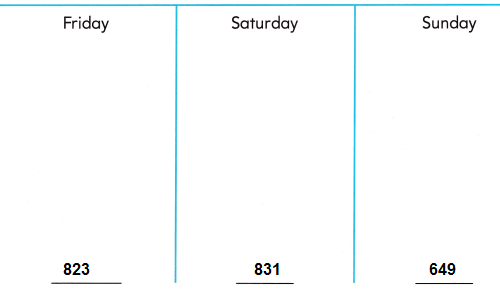Explanation:
From the above data On Friday, 823 people went to the fair.
On Saturday, 831 people went to the fair.
On Sunday, 649 people went to the fair.
By comparing the 3 days data Sunday is the least number of people at the fair and
Friday were the greatest number of people at the fair.

Math Talk
Mathematical Processes

Explain how you compared to find the least and greatest numbers.
Place value is compared to find the least and greatest numbers.
We consider a number greatest by arranging the given digits in descending order and similarly we consider a number least by arranging the digits in ascending order.

Model and Draw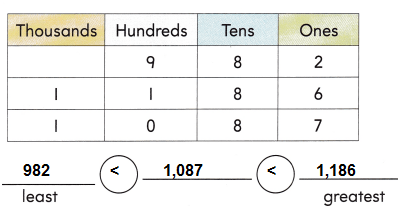Explanation:
We consider a number greatest by arranging the given digits in descending order and similarly we consider a number least by arranging the digits in ascending order.
Place value is compared to find the least and greatest numbers.

Share and Show

Compare the digits. Write the numbers in order.

Question 1.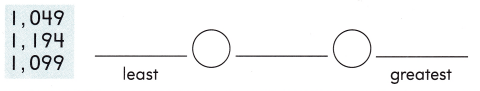Explanation:
We consider a number greatest by arranging the given digits in descending order and similarly we consider a number least by arranging the digits in ascending order.
Place value is compared to find the least and greatest numbers.

Question 2.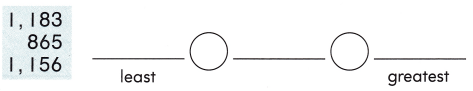Explanation:
When we compare numbers, check if both the numbers are having the same number of digits. If a number has more digits, then it is greater than the other number.
when two numbers are having the same number of digits,
we need to check the digit at the leftmost place, whichever is greater.
But if the left-most digit of both the numbers is the same,
then we need to check with the second left-most digit or so on.
Thus, in this way, we can find the greater or smaller number.

Question 3.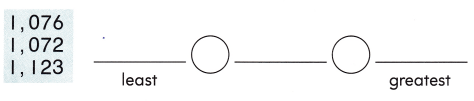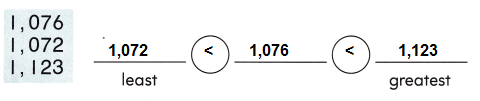Explanation:
When we compare numbers, check if both the numbers are having the same number of digits. If a number has more digits, then it is greater than the other number.
when two numbers are having the same number of digits,
we need to check the digit at the leftmost place, whichever is greater.
But if the left-most digit of both the numbers is the same,
then we need to check with the second left-most digit or so on.
Thus, in this way, we can find the greater or smaller number.

Problem Solving

Compare the digits. Write the numbers in order.

Question 4.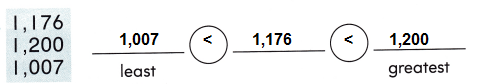Explanation:
When we compare numbers, check if both the numbers are having the same number of digits. If a number has more digits, then it is greater than the other number.
when two numbers are having the same number of digits,
we need to check the digit at the leftmost place, whichever is greater.
But if the left-most digit of both the numbers is the same,
then we need to check with the second left-most digit or so on.
Thus, in this way, we can find the greater or smaller number.

Question 5.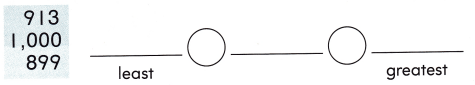Explanation:
When we compare numbers, check if both the numbers are having the same number of digits. If a number has more digits, then it is greater than the other number.
when two numbers are having the same number of digits,
we need to check the digit at the leftmost place, whichever is greater.
But if the left-most digit of both the numbers is the same,
then we need to check with the second left-most digit or so on.
Thus, in this way, we can find the greater or smaller number.

Use the numbers in the box to complete the sentences.Question 6.
___________ is greater than 1,100.
1,103 is greater than 1,100.
Explanation:
Arrange the above given numbers in ascending or descending order,
according to their place values, then we know 1,103 is greater than 1,100.

Question 7.
981 is less than _________.
981 is less than 1,047
Explanation:
When we compare numbers, check if both the numbers are having the same number of digits. If a number has more digits, then it is greater than the other number.
Question 8.
1,000 is greater than ___________.
1,000 is greater than 850
Explanation:
When we compare numbers, check if both the numbers are having the same number of digits. If a number has more digits, then it is greater than the other number.

Question 9.
H.O.T. At 3 schools, there are 972 students, 1,014 students, and 1,050 students. Write the numbers in order from greatest to least.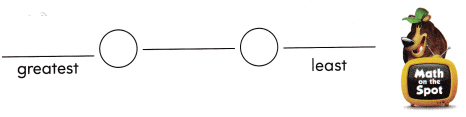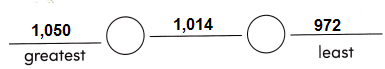Explanation:
Arrange 3 school students data in ascending or descending order.
When we compare numbers, check if both the numbers are having the same number of digits. If a number has more digits, then it is greater than the other number.
when two numbers are having the same number of digits,
we need to check the digit at the leftmost place, whichever is greater.
But if the left-most digit of both the numbers is the same,
then we need to check with the second left-most digit or so on.
Thus, in this way, we can find the greater or smaller number.

Question 10.
Multi-Step The number of shells Mary has is less than 1,034 but greater than 998.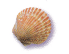How many shells might she have?
_________ shells
Explanation:
By comparing the greatest and smallest numbers,
Mary has 1,000 shells, because 1,000 is less than 1,034 but greater than 998.

Question 11.
Analyze At a coral reef, scientists counted 952 clownfish and some other fish.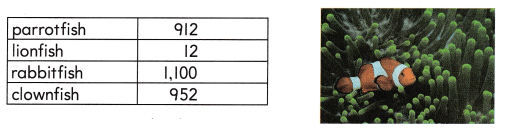Which of the following is true?
(A) There were more clownfish than rabbitfish.
(B) There were more clownfish than parrotfish.
(C) There were more lionfish than clownfish.
Option (B)
Explanation:
Number of clownfish = 952
Number of parrotfish = 912
Compare both the fishes according to their place values
952 > 912
Hence, There were more clownfish than parrotfish. statement is true.

Question 12.
Reasoning There are 874 white shells on the beach. There are fewer pink shells than white shells. How many pink shells could there be?
(A) 1,000
(B) 714
(C) 941
Option (B)
Explanation:
874 white shells
From the above options 1,000 and 941 are greater than 714
So, 714 pink shells on the beach,
but 714 pink shells are less than 874 white shells.
874 > 714
Question 13.
TEXAS Test Prep Which could be the unknown number?
741 < 1,062 < __________
(A) 1,029
(B) 1,068
(C) 895
Option (B)
Explanation:
From the above options 1,068 is the unknown number.
As, the other numbers 1,029 and 895 are less than 1,062.
741 < 1,062 < 1,068

TAKE HOME ACTIVITY • Have your child explain how he or she solved one of the exercises in the lesson.
Yes, by using effective problem solving techniques with concepts will help children doing with interest all exercises in the lesson.

Texas Go Math Grade 2 Lesson 3.4 Homework and Practice Answer Key

Compare the digits. Write the numbers in order.

Question 1.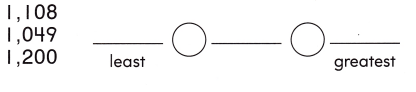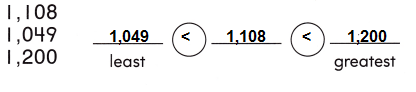Explanation:
When we compare numbers, check if both the numbers are having the same number of digits. If a number has more digits, then it is greater than the other number.
when two numbers are having the same number of digits,
we need to check the digit at the leftmost place, whichever is greater.
But if the left-most digit of both the numbers is the same,
then we need to check with the second left-most digit or so on.
Thus, in this way, we can find the greater or smaller number.

Use the numbers in the box to complete the sentences.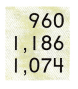Question 2.
1,061 is less than __________.
1,061 is less than 1,074
Explanation:
Arrange the above given numbers in ascending or descending order,
according to their place values and compare with the given number in the problem.
So, 1,061 is less than 1,074.

Question 3.
1,000 is greater than __________.
1,000 is greater than 960
Explanation:
Arrange the above given numbers in ascending or descending order,
according to their place values and compare with the given number in the problem.
So, 1,103 is greater than 1,100.

Question 4.
________ is greater than 1,000.
1074 is greater than 1,000.
Explanation:
Arrange the above given numbers in ascending or descending order,
according to their place values and compare with the given number in the problem.
So, 1,074 is greater than 1,000.

Problem Solving

Solve the problem.

Question 5.
Multi-Step The number of stickers Robert has is less than 1,145 but greater than 1,100. How many stickers might he have?
Explanation:
The number of stickers Robert has is less than 1,145 but greater than 1,100.
1,100 < 1,120 < 1,145
So, 1,120 stickers might Robert have.

Lesson Check

Question 6.
Which could be the unknown number?
541 < 1,074 < __________
(A) 378
(B) 1,088
(C) 1,056
Option (B)
Explanation:
From the above options 1,088 is the unknown number.
As, the other numbers 1,056 and 378 are less than 1,088.
So, after 1,074 we get 1,088.
541 < 1,074 < 1,088

Question 7.
There are 475 red crayons in a bucket. There are fewer green crayons than red crayons. How many green crayons could there be?
(A) 461
(B) 1,000
(C) 525
Option (A)
Explanation:
There are 475 red crayons in a bucket.
There are fewer green crayons than red crayons.
So, from the above options 461 is less than 475.
461 < 475

Question 8.
There are 962 small paper clips. There are more large paper clips than small paper clips. How many large paper clips could there be?
(A) 759
(B) 800
(C) 1,130
Option(C)
Explanation:
There are 962 small paper clips.
There are more large paper clips than small paper clips.
So, from the above options 1,130 is greater than 962.
962 < 1,130

Question 9.
The chart shows the number of crayons in a bin.Which of the following is true?
(A) There are more blue crayons than green crayons
(B) There are more yellow crayons than red crayons.
(C) There are more green crayons than red crayons.